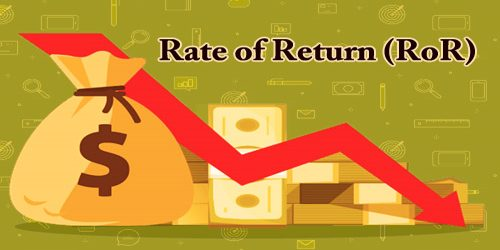Finance

# Rate of Return (RoR)The Rate of Return (RoR) is the amount we receive after the cost of an initial investment, calculated in the form of a percentage. The percentage can be reflected as a positive, which is considered a gain or profit. When the percentage is negative, it reflects a loss.

In finance, return is a profit on an investment. It comprises any change in value of the investment, and/or cash flows which the investor receives from the investment, such as interest payments or dividends. It may be measured either in absolute terms (e.g., dollars) or as a percentage of the amount invested. The latter is also called the holding period return. A loss instead of a profit is described as a negative return, assuming the amount invested is greater than zero.

A rate of return (RoR) can be applied to any investment vehicle, from real estate to bonds, stocks, and fine art. RoR works with any asset provided the asset is purchased at one point in time and produces cash flow at some point in the future. Investments are assessed based, in part, on past rates of return, which can be compared against assets of the same type to determine which investments are the most attractive. Many investors like to pick a required rate of return before making an investment choice.

Calculating the rate of return provides important information that can be used for future investments. For example, if we invested in a stock that showed a substantial gain after several months of performance, we may decide to purchase more of that stock. If the stock showed a continual loss, it may be wise to conduct research to find a better-performing stock.

Another advantage of calculating the rate of return is that it allows us to gauge our investment and decision-making skills. Investments that create a gain or profit are great. However, if we continually make investments at a loss, then we may want to change our investment strategies. A great attribute of successful business people knows how and when to make investments, as knows when to change strategies. With a firm grasp of calculating the rate of return, we can manage and monitor our investments at various stages to determine the outcome of our investments. This leads to a higher level of confidence and the skills necessary to be a savvy investor.

The formula to calculate the Rate of Return (RoR) is:

Rate of return = [(Current value − Initial value) / Initial value] ×100

Remember, the outcome is always reflected as a percentage, so the formula requires us to multiply by 100 to get the percentage. If this percentage is a positive number, then we have a profit or gain on our investment. If the percentage is a negative number, then we have a loss on the investment.

Rates of Return (RoR) are useful for making investment decisions. For nominal risk investments such as savings accounts or Certificates of Deposit, the investor considers the effects of reinvesting/compounding on increasing savings balances overtime to project expected gains into the future. For investments in which capital is at risks, such as stock shares, mutual fund shares, and home purchases, the investor also takes into consideration the effects of price volatility and risk of loss.

Ratios typically used by financial analysts to compare a company’s performance over time or compare performance between companies include return on investment (ROI), return on equity, and return on assets. A return may be adjusted for inflation. When the return is adjusted for inflation, the resulting return in real terms measures the change in purchasing power between the start and the end of the period. Any investment with a nominal annual return (i.e. unadjusted annual return) less than the annual inflation rate represents a loss of value in real terms, even when the nominal annual return is greater than 0%, and the purchasing power at the end of the period is less than the purchasing power at the beginning.

The rate of return (RoR) calculations for stocks and bonds is slightly different. Assume an investor buys a stock for \$60 a share, owns the stock for five years, and earns a total amount of \$10 in dividends. If the investor sells the stock for \$80, his per-share gain is \$80 – \$60 = \$20. In addition, he has earned \$10 in dividend income for a total gain of \$20 + \$10 = \$30. The rate of return for the stock is thus a \$30 gain per share, divided by the \$60 cost per share, or 50%.

On the other hand, consider an investor that pays \$1,000 for a \$1,000 par value 5% coupon bond. The investment earns \$50 in interest income per year. If the investor sells the bond for \$1,100 in premium value and earns \$100 in total interest, the investor’s rate of return is the \$100 gain on the sale, plus \$100 interest income divided by the \$1,000 initial cost or 20%.

The rate of return (RoR) which an investor requires from a particular investment is called the discount rate and is also referred to as the (opportunity) cost of capital. The higher the risk, the higher the discount rate (rate of return) the investor will demand from the investment.

Information Sources: